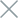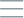• Home
• Tags
• Series
•# Binary Search Algorithm in C++ - Explanation, Example, and Code

The binary search algorithm is a more efficient way of searching for a specific element in a sorted list. Unlike linear search, which checks elements sequentially, binary search divides the list into halves and compares the target element with the middle element. This process is repeated until the target element is found or the search range becomes empty.

## How It Works

2. Find the middle element of the current search range.
3. Compare the middle element with the target value.
4. If the middle element equals the target value, the search is successful.
5. If the middle element is greater than the target, search in the left half of the range.
6. If the middle element is smaller than the target, search in the right half of the range.
7. Repeat steps 2-6 until the target element is found or the search range becomes empty.

## Example

Let's consider a sorted list of integers and want to find the number 34 using binary search.

Sorted List: {12, 23, 34, 45, 56, 67, 89, 90}

2. Middle element: 56 (position 4). Compare with 34.
3. 56 is greater than 34. Search in the left half.
4. New middle element: 23 (position 1). Compare with 34.
5. 23 is smaller than 34. Search in the right half.
6. New middle element: 45 (position 3). Compare with 34.
7. 45 is greater than 34. Search in the left half.
8. New middle element: 34 (position 2). Target found.

## Example Code in C++

``````#include <iostream>
#include <vector>

int binarySearch(const std::vector<int>& arr, int target) {
int left = 0;
int right = arr.size() - 1;

while (left <= right) {
int mid = left + (right - left) / 2;

if (arr[mid] == target) {
return mid;
} else if (arr[mid] < target) {
left = mid + 1;
} else {
right = mid - 1;
}
}

return -1;
}

int main() {
std::vector<int> numbers = {12, 23, 34, 45, 56, 67, 89, 90};
int target = 34;

int result = binarySearch(numbers, target);

if (result != -1) {
std::cout << "Element " << target << " found at position " << result << std::endl;
} else {
std::cout << "Element " << target << " not found in the array" << std::endl;
}

return 0;
}
``````

In the given example, the `binarySearch` function is used to find the number 34 in a sorted list of integers. The result will be the position of 34 in the list (positions start from 0) or -1 if the number is not found.